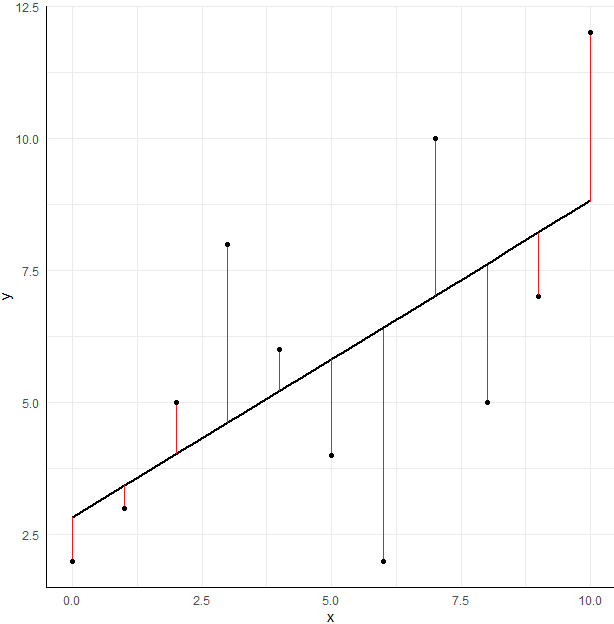# Residuals in multivariate analysis

Modified on Mon, 16 Nov 2020 at 02:09 PM

# What are residuals in multivariate analysis?

The aim of a model in multivariate analysis is to assess the relationship between a response variable and explanatory variables. It is wished that the explanatory variables could explain the totality of the response observed. In fact, it never happens.

Thus, the explanatory variables representing the explanatory part of the model, the residuals represent the part of the response that is not explained by the explanatory variables. The residuals of the model are mathematically represented by the error term Ɛ. This term could be positive or negative, laying the model to over or underestimate the value of the response variable. To counterbalance this bias, the intercept term is also computed in multivariate analysis.The residuals are represented in this scatterplot by the red lines connecting the points to the regression line.

For more details, see these books:

Montgomery D.C., Peck E.A., Vining G.G. Introduction to linear regression analysis. Wiley. 5th edition. 2012

Harrell F.E. Regression modeling strategies with applications to linear models, logistic and ordinal regression, and survival analysis. Springer. 2015.